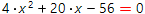Quadratic equation is equation of the form:

a∙x 2 + b∙x + c = 0

On the basis of this calculator is discriminant approach. However, in some special cases (when one or more coefficients are equal to zero) more simple approach is used. So, this calculator tests input equation and choose the most simple solution. Step by step solution is also available for free.

Coefficients a, b and c of quadratic equation can be decimal numbers, fractions and even parameters. The leading coefficient at x2 can't be zero. The equation should be entered in its natural form.

On the basis of the discriminant sign, the quadratic solution can have only real or two complex conjugate roots (if all the coefficients are real). Our calculator automatically handles all these cases.